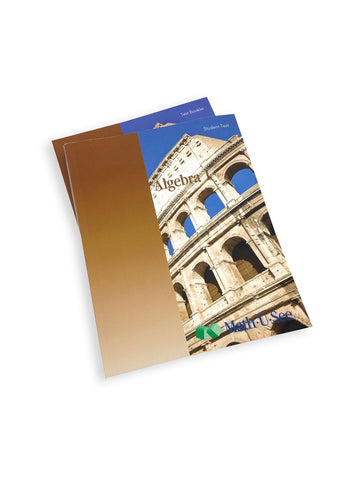# Math-U-See Algebra 1 Student Pack

• \$ 48.00

Algebra 1 Student Pack contains Student Workbook and Algebra 1 Tests.

Graphing, simultaneous equations, exponents, polynomials, unit multipliers, and more.

Major Concepts and Skills Include:

• Graphing linear equations and inequalities
• Describing linear equations in slope-intercept and standard form
• Solving systems of equations using graphing, substitution, and elimination
• Expanding understanding of exponents
• Solving basic quadratic equations with factoring
• Using unit multipliers to convert between units of measure